Download Presentation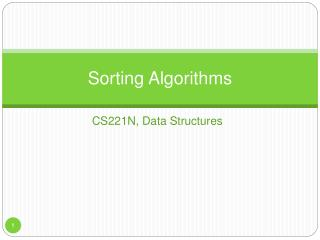Sorting Algorithms

# Sorting Algorithms - PowerPoint PPT Presentation

Sorting Algorithms. CS221N, Data Structures. Sorting in Databases. Many possibilities Names in alphabetical order Students by grade Customers by zip code Home sales by price Cities by population Countries by GNP Stars by magnitude. Sorting and Searching.I am the owner, or an agent authorized to act on behalf of the owner, of the copyrighted work described.
Download Presentation## Sorting Algorithms

An Image/Link below is provided (as is) to download presentation

Download Policy: Content on the Website is provided to you AS IS for your information and personal use and may not be sold / licensed / shared on other websites without getting consent from its author.While downloading, if for some reason you are not able to download a presentation, the publisher may have deleted the file from their server.

- - - - - - - - - - - - - - - - - - - - - - - - - - E N D - - - - - - - - - - - - - - - - - - - - - - - - - -
Presentation Transcript### Sorting Algorithms

CS221N, Data StructuresSorting in Databases
• Many possibilities
• Names in alphabetical order
• Students by grade
• Customers by zip code
• Home sales by price
• Cities by population
• Countries by GNP
• Stars by magnitudeSorting and Searching
• We saw with arrays they could work in tandem to improve speed
• What was the search method that required sorting an array?
• How much faster was the search?
• But that means nothing if sorting takes forever!
• For this and its usefulness, sorting algorithms have been heavily researched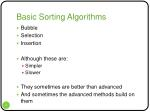Basic Sorting Algorithms
• Bubble
• Selection
• Insertion
• Although these are:
• Simpler
• Slower
• They sometimes are better than advanced
• And sometimes the advanced methods build on them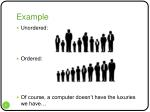Example
• Unordered:
• Ordered:
• Of course, a computer doesn’t have the luxuries we have…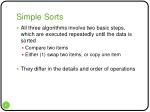Simple Sorts
• All three algorithms involve two basic steps, which are executed repeatedly until the data is sorted
• Compare two items
• Either (1) swap two items, or copy one item
• They differ in the details and order of operations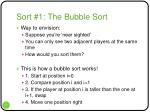Sort #1: The Bubble Sort
• Way to envision:
• Suppose you’re ‘near sighted’
• You can only see two adjacent players at the same time
• How would you sort them?
• This is how a bubble sort works!
• 1. Start at position i=0
• 2. Compare position i and i+1
• 3. If the player at position i is taller than the one at i+1, swap
• 4. Move one position right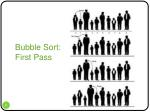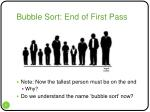Bubble Sort: End of First Pass
• Note: Now the tallest person must be on the end
• Why?
• Do we understand the name ‘bubble sort’ now?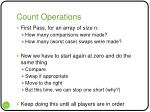Count Operations
• First Pass, for an array of size n:
• How many comparisons were made?
• How many (worst case) swaps were made?
• Now we have to start again at zero and do the same thing
• Compare
• Swap if appropriate
• Move to the right
• But this time, we can stop one short (why?)
• Keep doing this until all players are in orderLet’s do an example ourselves…
• Use a bubble sort to sort an array of ten integers:
• 30 45 8 204 165 95 28 180 110 40How many operations total then?
• First pass: n-1 comparisons, n-1 swaps
• Second pass: n-2 comparisons, n-2 swaps
• Third pass: n-3 comparisons, n-3 swaps
• (n-1)th pass: 1 comparison, 1 swap
• Then it’s sorted
• So we have (worst case):
• (n-1)+(n-2)+….+1 comparisons = n(n+1)/2 = 0.5(n2+n)
• (n-1)+(n-2)+….+1 comparisons = n(n+1)/2 = 0.5(n2+n)
• Total: 2*(0.5(n2+n)) = n2+n = O(n2)Java Implementation
• Let’s go through the example together on page 85
• Zero in on the bubble sort method
• Note how simple it is (4 lines)!
• Also note: the outer loop goes backwards!
• Why is this more convenient? Look where else this loop counter is used.
• We also have a function for swappingInvariants
• Algorithms tend to have invariants, i.e. facts which are true all the time throughout its execution
• In the case of the bubble sort, what is always true is….
• Worth thinking about when trying to understand algorithmsSort #2: Selection Sort
• Purpose:
• Improve the speed of the bubble sort
• Number of comparisons: O(n2)
• Number of swaps: O(n)
• We’ll go back to the baseball team…
• Now, we no longer compare only players next to each other
• Rather, we must ‘remember’ heights
• So what’s another tradeoff of selection sort?What’s Involved
• Make a pass through all the players
• Find the shortest one
• Swap that one with the player at the left of the line
• At position 0
• Now the leftmost is sorted
• Find the shortest of the remaining (n-1) players
• Swap that one with the player at position 1
• And so on and so forth…Count Operations
• First Pass, for an array of size n:
• How many comparisons were made?
• How many (worst case) swaps were made?
• Now we have to start again at position one and do the same thing
• Find the smallest
• Swap with position one
• Keep doing this until all players are in orderLet’s do an example ourselves…
• Use a selection sort to sort an array of ten integers:
• 30 45 8 204 165 95 28 180 110 40How many operations total then?
• First pass: n-1 comparisons, 1 swap
• Second pass: n-2 comparisons, 1 swap
• Third pass: n-3 comparisons, 1 swap
• (n-1)th pass: 1 comparison, 1 swap
• Then it’s sorted
• So we have (worst case):
• (n-1)+(n-2)+….+1 comparisons = n(n-1)/2 = 0.5(n2 - n)
• 1+1+….+1 comparisons = n-1
• Total: 0.5(n2 - n) + (n – 1) = 0.5n2 + 0.5n - 1 = O(n2)Implementation – page 92
• Let’s go through the function together.
• Things to watch:
• A bit more complex than bubble (6 lines)
• A bit more memory (one extra integer)A method of an array class…
• See page 93, we’ll go through it.Sort #3: Insertion Sort
• In most cases, the best one…
• 2x as fast as bubble sort
• Somewhat faster than selection in MOST cases
• Slightly more complex than the other two
• More advanced algorithms (quicksort) use it as a stageProceed..
• A subarray to the left is ‘partially sorted’
• The player immediately to the right is ‘marked’.
• The ‘marked’ player is inserted into the correct place in the partially sorted array
• Remove first
• Marked player ‘walks’ to the left
• Shift appropriate elements until we hit a smaller oneLet’s do an example ourselves…
• Use a insertion sort to sort an array of ten integers:
• 30 45 8 204 165 95 28 180 110 40Count Operations
• First Pass, for an array of size n:
• How many comparisons were made?
• How many swaps were made?
• Were there any? What were there?
• Now we have to start again at position two and do the same thing
• Move the marked player to the correct spot
• Keep doing this until all players are in orderHow many operations total then?
• First pass: 1 comparison, 1 copy
• Second pass: 2 comparisons, 2 copies
• Third pass: 3 comparisons, 3 copies
• (n-1)th pass: n-1 comparison, n-1 copies
• Then it’s sorted
• So we have (worst case):
• (n-1)+(n-2)+….+1 comparisons = n(n-1)/2 = 0.5(n2- n)
• (n-1)+(n-2)+….+1 copies = n(n-1)/2 = 0.5(n2- n)
• Total: 2*(0.5(n2+n)) = n2- n = O(n2)Why are we claiming it’s better than selection sort?
• A swap is an expensive operation. A copy is not.
• To see this, how many copies are required per swap?
• Selection/bubble used swaps, insertion used copies.
• Secondly, if an array is sorted or almost sorted, insertion sort runs in approximately O(n) time. Why is that?
• How many comparisons would you need per iteration? And how many copies?
• Bubble and selection would require O(n2) comparisons even if the array was completely sorted initially!Implementation
• We’ll write the function (pages 99-100) together
• Use the control flow on the previous slideAnd finally…
• Encapsulate the functionality within an array class (p. 101-102)Invariant of Insertion Sort
• At the end of each pass, the data items with indices smaller than __________ are partially sorted.Sorting Objects
• Let’s modify our insertion sort to work on a Person class, which has three private data members:
• lastName (String)
• firstName (String)
• age (int)
• What do we need for something like insertion sort to work?
• How do we define that?Lexicographic Comparison
• For Java Strings, you can lexicographically compare them through method compareTo():
• s1.compareTo(s2);
• Returns an integer
• If s1 comes before s2 lexicographically, returns a value < 0
• If s1 is the same as s2, return 0
• If s1 comes after s2 lexicographically, returns a value > 0Stable Object Sorts
• Suppose you can have multiple persons with the same last name.
• Now given this ordering, sort by something else (first name)
• A stable sort retains the first ordering when the second sort executes.
• All three of these sorts (bubble, selection, insertion) are stable.Sort Comparison: Summary
• Bubble Sort – hardly ever used
• Too slow, unless data is very small
• Selection Sort – slightly better
• Useful if: data is quite small and swapping is time-consuming compared to comparisons
• Insertion Sort – most versatile
• Best in most situations
• Still for large amounts of highly unsorted data, there are better ways – we’ll look at them
• Memory requirements are not high for any of these